Mathematical and Physical Journal
for High Schools
Issued by the MATFUND Foundation
 Already signed up? New to KöMaL?

# KöMaL Problems in Informatics, May 2014

Show/hide problems of signs:## Problems with sign 'I'

Deadline expired on June 10, 2014.

I. 349. The first figure of this exercise shows a rectangular area consisting of $\displaystyle N\times M$ ($\displaystyle 2\le N,M\le 50$) tower blocks viewed from above. The horizontal cross sections of the towers are identical squares. The number of levels of a tower block varies between 1 and 9; the value 0 denotes a square-shaped park area. The figure Szomszédság'' (neighborhood) shows that a particular square has 4 neighboring squares by definition. On each level of each tower block there is exactly one flat.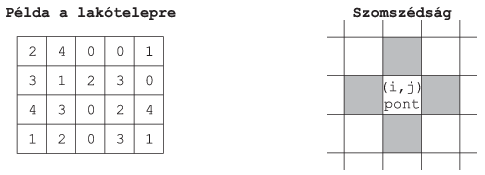Create your program i349 to answer the following questions by using data from the file telep.txt. Before writing any result to the screen, the number of the actual task (for example, Task #3:) should be displayed. If the user is prompted to enter any data, then the type of this requested input should also be shown. Diacritical marks in the output may be omitted.

The first line of the file telep.txt contains the values of $\displaystyle N$ and $\displaystyle M$. The following $\displaystyle N$ lines describe the number of levels of each tower block or the parks, see the example.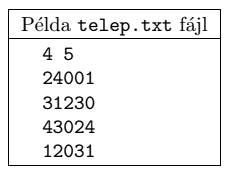1. Read the data from the file telep.txt to solve the following tasks.

2. Determine and display to 2 decimal digits the percentage of the park area relative to the total area.

3. The view from a window is important to many people. By definition, one can see out of a window of a given flat in a particular direction (vertical or horizontal, if viewed from above) as long as the neighboring flats are lower than the actual one. Display the number of flats with the property that there is a (vertical or horizontal) direction such the border of the rectangular area occupied by the tower blocks can be seen from that window of the flat.

4. Some customers are interested not only in the view, but also in being close to a park area or to the border of the rectangular area. Prompt a user to enter a level number, then display the total number of flats on that level with the property that one has a view in some direction, and how many of these flats are adjacent to a park square or the rectangle border. The result should be displayed in the following format: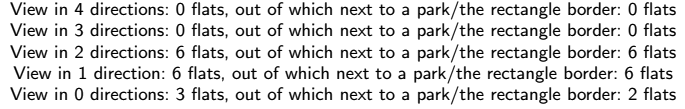5. From an architectural point of view, the visibility of the whole set of tower blocks is also important. For each row and column of the rectangle, you should determine the number of visible tower blocks if viewed from the east or west directions (for rows), or from the north or south directions (for columns). A tower block is obscured by another tower block of the same size or higher. The results should be written to the file latvany.txt in the following format: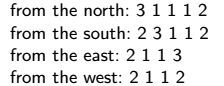6. Some people would like to live close to a large park area. Read the position of a square in a (row, column) format relative to the top left corner of the rectangle, and display whether there is a park there. If yes, give the number of squares the park occupies.

The source code (i349.pas, i349.cpp, ...) of your program and a brief documentation (i349.txt, i349.pdf, ...) of your solution should be submitted, also specifying the name of the developer environment to use for compiling your source.

(10 pont)

solution (in Hungarian), statistics

I. 350. We would like to store the data and process the results of a football championship with 12 football teams by using a spreadsheet application. You can use 3 sheets, Csapatok (teams), Körmérkőzés (tournament) and Eredmények (results). Auxiliary computations can be performed below or to the right of the data and results of the present task (by leaving one blank row or column). Macros or your own programs should not be used.

1. You should write some team names into cells A1:A12 of the sheet Csapatok (one can decide what teams to invite to the championship).

2. You should create 2 tables on the sheet Körmérkőzés according to the example. The left table should contain the number of scored goals and received goals during each match, whereas the right table should contain the same information in a form scored $\displaystyle \text{goals} : \text{received}$ goals''. You should fill the left table with random integers between 0 and 8 as in the example. A $\displaystyle -1$ value should be present in the table where no match can take place. Similarly, you should put $\displaystyle -1$ values in the appropriate cells in the right table. By using conditional formatting, both the text and background colors of a cell containing $\displaystyle -1$ should be set to grey.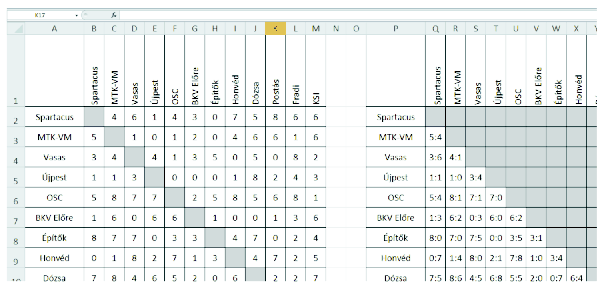3. You should give the team names in the range A2:A13 of the sheet Eredmény. Next to it, in column B you should compute the scored goals, in column C the received ones, and in column D the goal difference.

4. Columns E, F and G should contain for each team the number of won, lost or tied matches.

5. Column H should contain the total number of points for each team. Won, tied and lost matches are worth 2, 1 and 0 points, respectively.

6. Column I should contain the team ranking based on the number of team points. If the team points are equal, the ranking is based on a better goal difference.

7. The header of the sheet Eredmény in cells B1:I1 should be set according to the previous tasks.

You can get the maximal number of points for this task only if you use copyable formulae to describe regions containing the same type of data. You should use the same simple border type as in the example in your solutions to the above tasks or when separating your auxiliary computations. The spreadsheet i350 (.xls, .xlsx, ...) containing the solution should be submitted.

(10 pont)

solution (in Hungarian), statistics

I. 351. In Exercise C. 1207. (see our January 2014 issue) one had to verify a proposition stating that the ratio of the area of certain two figures is greater than $\displaystyle 1:2$ for any integer $\displaystyle n\ge 5$. Now we consider the following modification: find all real numbers $\displaystyle n>1$ such that the area ratio is exactly $\displaystyle 1:2$. By using your own program or any freely available software, determine the value of $\displaystyle n$ to 6 decimal digits.

You should submit a text file i351.txt, i351.pdf, ...containing the value of $\displaystyle n$ to the requested precision, a brief description of the solution method, a link to the software you chose and the files used in the solution, or the source code of your own program and the necessary information to run the code.

(10 pont)

solution (in Hungarian), statistics## Problems with sign 'S'

Deadline expired on June 10, 2014.

S. 90. A cave consists of some chambers and passages connecting the chambers. A secret agent hid a valuable gem in one of the chambers. We would like to recover this treasure. The following information is known about the cave: it has $\displaystyle 1\le N\le 200\;000$ chambers, and there is exactly one path between any two chambers. We would like to find the gem without entering the cave by asking questions from the secret agent about the gem's location. The form of a question is always Is the gem located in the $\displaystyle i^\mathrm{th}$ chamber?'' The agent answers this question, and if the answer is No'', then also tells us the proper direction to find the gem. The difficulty lies in the fact that the agent wants to avoid any unnecessary questioning. So your task is to minimize the number of questions you ask from the agent. The maximal number of points for this exercise can only be obtained if your program correctly determines the minimal number of questions to locate the gem even if one should ask the maximal possible number of questions. In other words, your goal is to give the minimum number of questions that guarantee the recovery of the gem.

Your program should read the value of $\displaystyle N$ from the first line of the standard input, then the next $\displaystyle N-1$ lines containing the map of the cave: each line contains a pair of integers $\displaystyle a_i$, $\displaystyle b_i$ representing a direct passage between chambers $\displaystyle a_i$ and $\displaystyle b_i$. Your solution, the minimal number of questions, should be written to the first and only line of the standard output. In the example, Példa bemenet'' and Példa kimenet'' are a sample input and the corresponding output.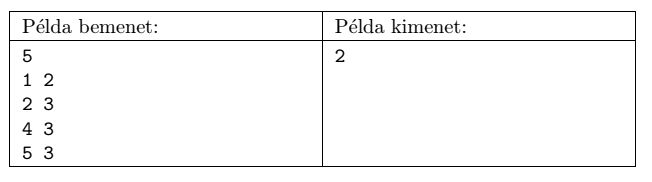Scoring and bounds. You can obtain 1 point for a brief and proper documentation clearly describing your solution. Nine further points can be obtained provided that your program solves any arbitrary valid input within 1 second of running time. We will run your program on several test cases. We will count it as a critical error if your program produces an output that is less than the necessary minimum. However, you can get partial points if your output is more than the optimum. Recall that the recovery of the gem must be guaranteed by asking the appropriate number of questions. Partial points can be obtained if your program yields a solution

$\displaystyle \bullet$ for $\displaystyle N\le 200$, or

$\displaystyle \bullet$ for $\displaystyle N\le 5000$.

The source code (s90.pas, s90.cpp, ...) without the .exe or any other auxiliary files generated by the compiler but with a short documentation (s90.txt, s90.pdf, ...), also describing which developer environment to use for compiling the source, should be submitted in a compressed file s90.zip.

(10 pont)

statistics

\$Var(Body)